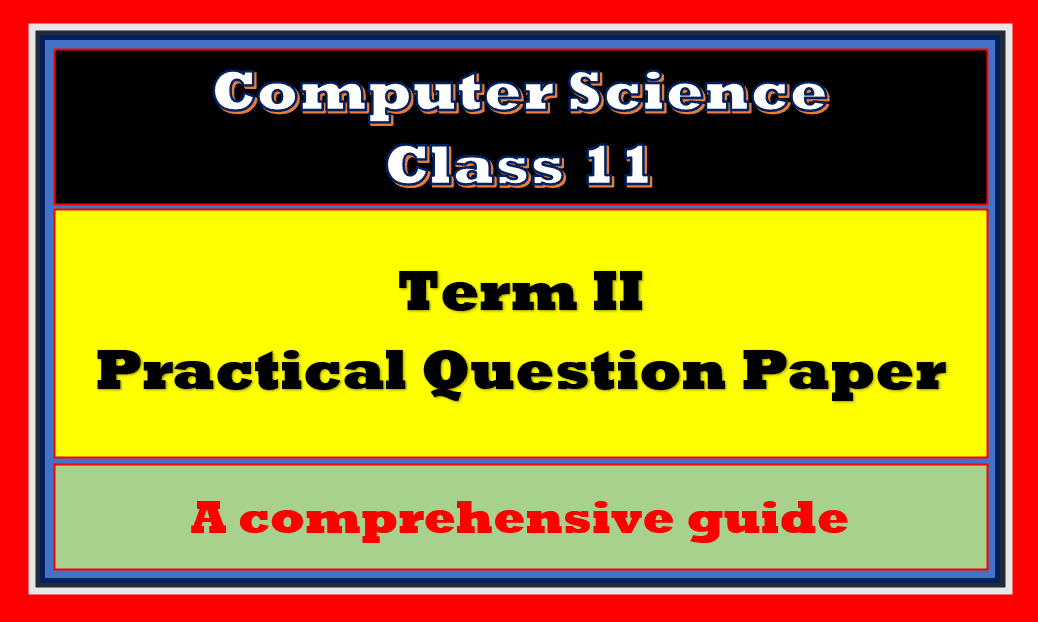In this article, I am going to discuss Class 11 CS Practical Paper 2023. The practical Exam in Session 2022-23 for class 11, will be conducted at the end of the year as Annual Exam. So let’s start it!

Topics Covered

## Topics Class 11 CS Practical Paper 2023

The following topics should be covered in the practical assessment.

1. Python Fundamentals
2. If else
3. Iteration (Loops)
4. Lists
5. Tuples
6. Dictionary
7. Introduction to Modules

The Class 11 CS Practical Paper 2023 has the following marks distribution:

So here we go for Class 11 CS Practical Paper 2023.

## Python Program for Class 11 CS Practical Paper

The question for Class 11 CS Practical Paper 2023 contains a python program from a List, tuple, or dictionary. I have taken the program from the dictionary part.

Create a dictionary named student and write a menu-driven program to do the following:

1. Show Record
3. Delete a student
4. Search record
5. Update record
6. Sort record
7. Exit

Solution:

``````n=int(input("How many stduents:"))
std={}
for i in range(n):
print("Enter details of student:",i+1)
name=input("Enter name of student:")
pn=int(input("Enter Percentage:"))
std[name]=pn
c=0
while c!=7:
print('''
1. Show record
3. Delete a student
4. Search record
5. Update record
6. Sort record
7. Exit
''')
if c==1:
for i in std:
print(i,":",std[i])
elif c==2:
print("Enter details of new customer:")
name=input("Enter name:")
pn=int(input("Enter Percentage:"))
std[name]=pn
elif c==3:
nm=input("Enter name to be deleted:")
f=std.pop(nm,-1)
if f!=-1:
print(f, " is deleted successfully.")
else:
elif c==4:
name=input("Enter Customer Name:")
if name in std:
print(name, " found in the record.")
else:
elif c==5:
name=input("Enter Customer Name:")
pn=int(input("Enter percentage to modify:"))
std[name]=pn
elif c==6:
l=sorted(std)
for i in l:
print(i,":",std[i])
elif c==7:
break
else:
print("Invalid Choice")``````

## Practical File Class 11 CS Practical Paper

The practical record file is one of the components of Class 11 CS Practical Paper 2023 and contains the regular records of python programs.

This practical file should be submitted either handwritten or hard copy. It contains a minimum of 20 programs based on the syllabus. If you are looking for Practical File Class 11 computer science, follow this link:

The next component of Class 11 CS Practical Paper 2023 is viva voce. Let’s discuss the viva voce.

## Viva Voce Class 11 CS Practical Paper 2023

In the viva voce oral questions can be asked from the practical file and the syllabus covered throughout the year. These questions can be any random questions asked by the examiner. So be prepared for those questions.

## Project and Viva Voce Class 11 CS Practical Paper 2023

The aim of the class project is to create something that is tangible and useful using Python list/tuple/dictionary.

This should be done in groups of two to three students and should be started by students at least 6 months before the submission deadline.

The aim here is to find a real-world problem that is worthwhile to solve. Students are encouraged to visit local businesses and ask them about the problems that they are facing.

For example, if a business is finding it hard to create invoices for filing GST claims, then students can do
a project that takes the raw data (list of transactions), groups the transactions by category, accounts for
the GST tax rates, and creates invoices in the appropriate format.

Students can be extremely creative here. They can use a wide variety of Python libraries to create user-friendly applications such as games, software for their school, software for their disabled fellow students, and mobile applications, of course, to do some of these projects, some additional learning is required; this should be encouraged. Students should know how to teach themselves.

The students should be sensitized to avoid plagiarism and violations of copyright issues while working on
projects. Teachers should take the necessary measures for this.

Watch this video for more understanding:

If you are looking for the PDF, here I am going to link the same as follows:

I hope this article Class 11 CS Practical Paper 2023 will help you to prepare for your practical exam. If you have any queries or doubt related to Class 11 CS Practical Paper 2023 feel free to ask in the comment section. Feel free to share your views, feedback and suggestions to improve the content.